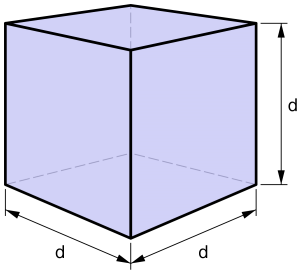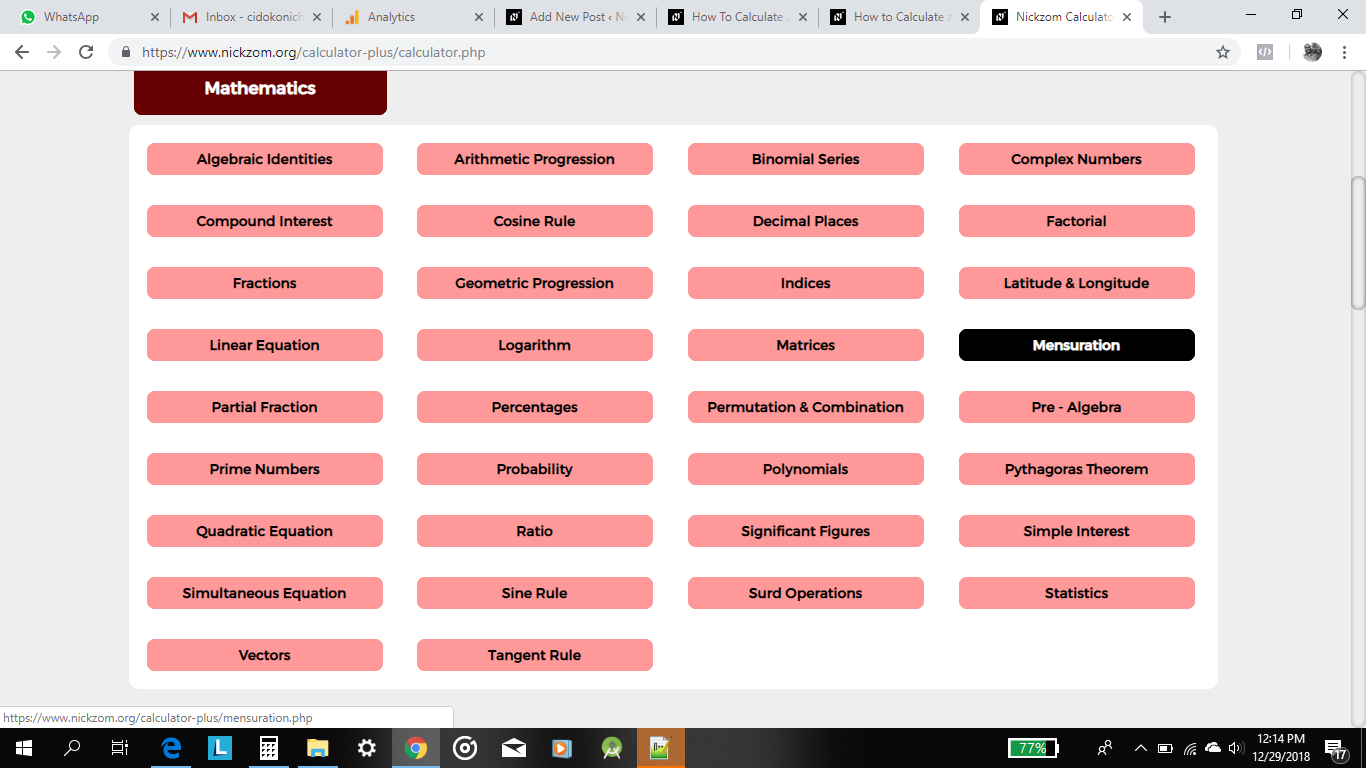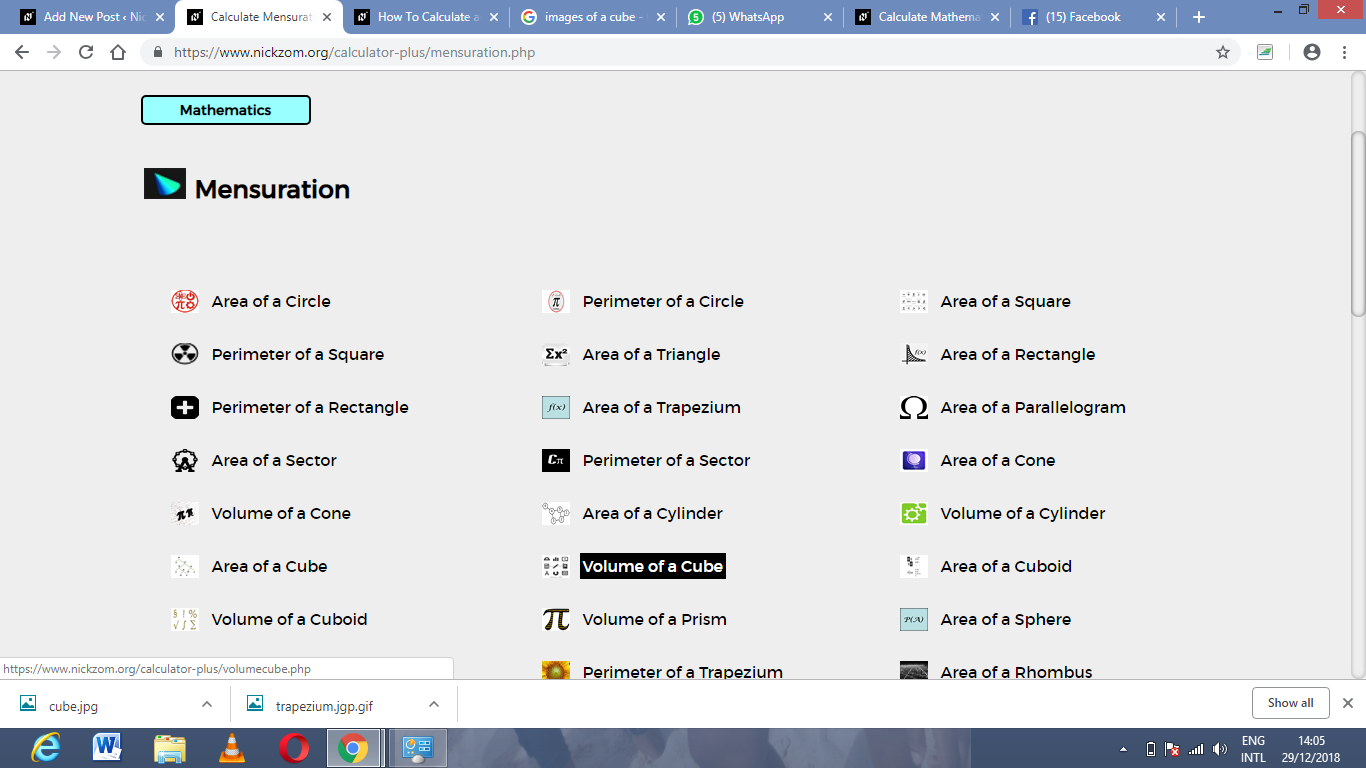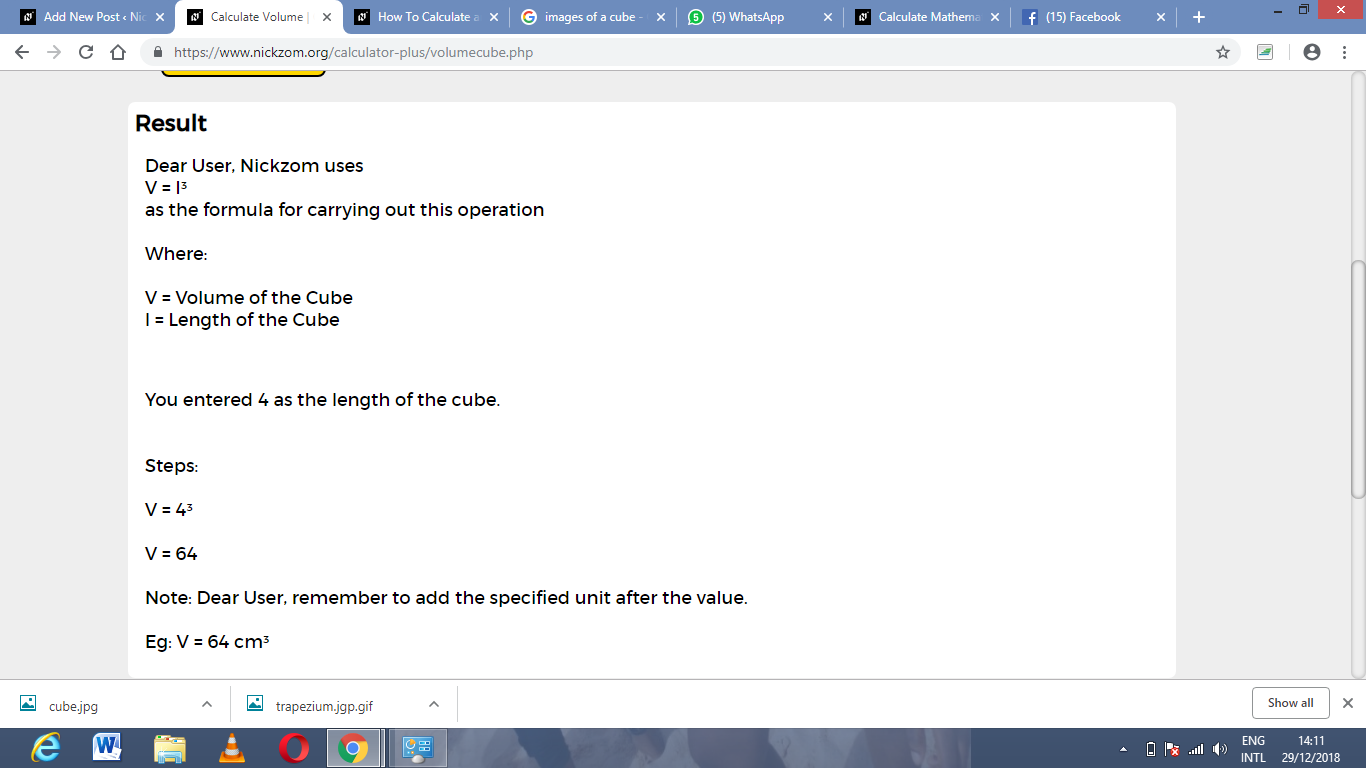# How to Calculate and Solve for the Volume and Length of a Cube | The Calculator EncyclopediaThe image above is a cube.

To compute the volume of a cube, one essential parameter is needed and this parameter is the length of the cube (l).

The formula for calculating the volume of a cube is;

V = l3

Where;

V = Volume of a cube
l = Length of a cube

Let’s solve an example:
Find the volume of a cube where the length of a cube is 4 cm.

This implies that;
l = length of the cube = 4 cm.

V = l3
V = 43
V = 64 cm3

Therefore, the volume of the cube is 64 cm3

Calculating the Length of a cube using the Volume of the cube.

The formula is l = 3√V

Where;

V = Volume of a cube
l = length of a cube

Let’s solve an example:
Find the length of a cube where the volume of the cube is 120 cm3

This implies that;
V = Volume of the cube = 120 cm3

l = 3√V
l = 3√120
l = 4.93

Therefore, the length of the cube is 4.93 cm.

Nickzom Calculator – The Calculator Encyclopedia is capable of calculating the volume of a cube.

To get the answer and workings of the area of a circle using the Nickzom Calculator – The Calculator Encyclopedia. First, you need to obtain the app.

You can get this app via any of these means:

To get access to the professional version via web, you need to register and subscribe for NGN 1,500 per annum to have utter access to all functionalities.
You can also try the demo version via https://www.nickzom.org/calculator

Once, you have obtained the calculator encyclopedia app, proceed to the Calculator Map, then click on Mensuration under the Mathematics sectionNow, click on Volume of a Cube under MensurationThe screenshot below displays the page or activity to enter your value, to get the answer for the volume of a cube accordingly with its respective parameter which is the length of the cube.Now, enter the value appropriately and accordingly for the parameter as required by the example above where the length of the cube is 4 cm.Finally, click on calculate;As you can see from the screenshot above, Nickzom Calculator – The Calculator Encyclopedia solves for the volume of a cube and presents the formula, workings and steps too.